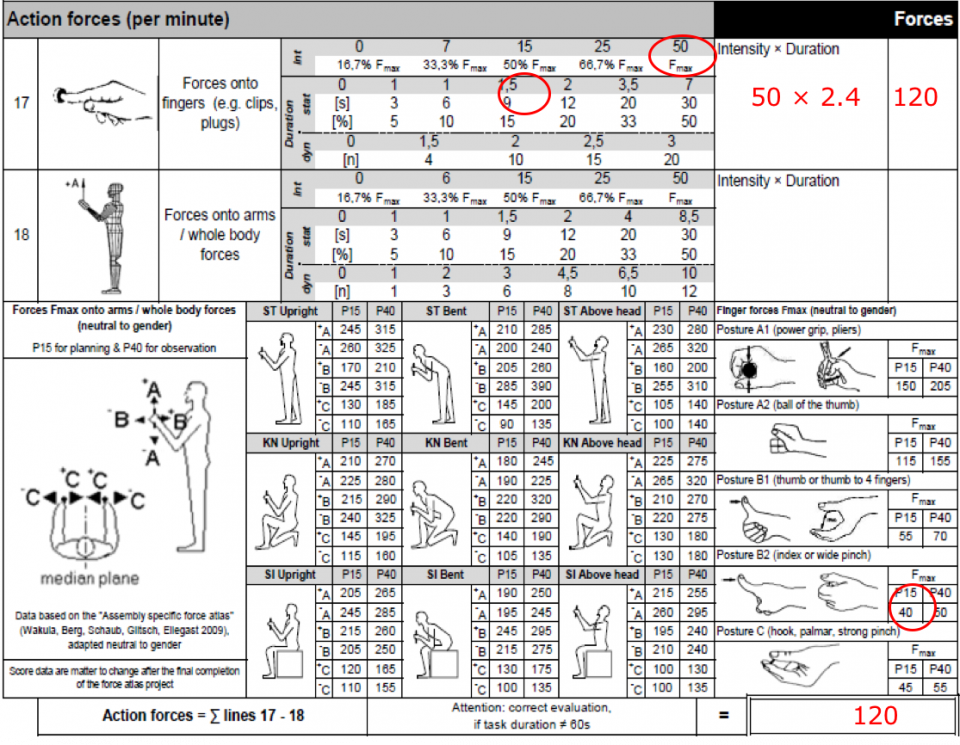# Solution

P1 Score

Intensity Points: 100% Fmax = 50 Points

Duration Points: 10 s/cycle = 1.7 Points

P1= I1 x D1 = 50 x 1 = 50 Points <- Worst Case

P2 Score

Intensity Points: 80% Fmax = 35 Points

Duration Points: 6 s/cycle = 1 Point

P2= I2 x D2 = 35 x 1 = 35 Points

Risk Score Calculation (R)

Intensity Points: P1

Intensity Points = 50 Points

Frequency = P1 Frequency x (1+P2/(P1+P2))= 10 x (1+35/(50+35))= 10 x (1+0.4)= 14 s/cycle

Duration Points = 2,4 Points

R = I x D = 50 x 2.4 = 120 Points